Last weekend, I shared some interesting properties of numbers with my kids.

The great thing about explaining something to a non-expert is that you have to actually understand the topic. (This is why making teaching universities and research universities the same actually makes sense.) If you hide behind a formalism, the explanation won’t work. Usually, this means that you didn’t understand why the formalism worked either.

This is why I thought “why are far away things smaller?” was such a great question. “Similar triangles” answers are brittle, and if a tiny error makes far away things come out bigger instead, you won’t catch yourself until you got to the end of the proof.

Some of the interesting properties of numbers are: that (n + 1)Ã—(n-1)=n_2-1: that the perfect squares (0,1,4,9,…) go up by successive odd numbers (1,3,5,…); and that the area of a triangular number (1+2+…+_n) has a closed form. These properties are easy to show using algebra, but to a child, the algebra doesn’t make sense.

Multiplication and division are grounded in visuospatial concepts, which is why these number theoretical results are easy to understand. What would a proof that stayed grounded in visuospatial concepts look like?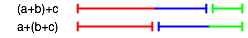and commutative: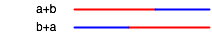## Multiplication is Commutative

The commutative law is that aÃ—b=bÃ—a. If a rectangle a high and b wide represents aÃ—b , this is the same as saying that rotation is area-preserving: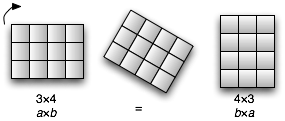Or, in the case of three factors, volume preserving: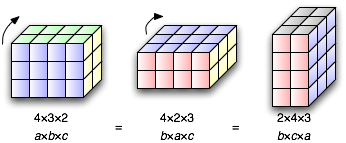## Distributive Law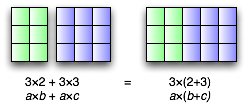## Associativity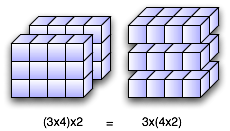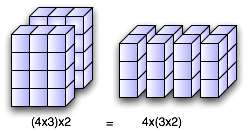## Difference of Squares

The perfect squares are 0, 1, 4, 9, 16, …. The differences between successive squares are the odd numbers: 1, 3, 5, 7, 9, ….

In algebra, this is because the n_2 terms in (_n+1)2=n_2+2_n+1 and in n_2 cancel, leaving 2_n+1. Here’s why this is true in geometry: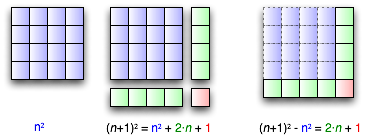## Product of Alternates

 1x1=1 0x2=0 2x2=4 1x3=3 3x3=9 2x4=8 4x4=16 3x5=15 5x5=25 4x6=24

The product of two numbers adjacent to a median, is one less than the square of the median. For example, 52=25, and 6Ã—4=25-1=24. Algebraically, this is because (n+1)(n-1)=_n_2-1. Geometrically: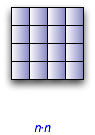!/images/2004/grounded-proofs/4x3.png! !/images/2004/grounded-proofs/3x3-4x3.png!

## Triangle Numbers

In closed form, the sum of the numbers from 1 to n is n(n+1)/2. One algebraic proof for this uses induction: substitute each of k+1 and k for n, and compute the difference.

There are two geometric proofs. The first requires two cases: one for even n, and one for odd. The second is simpler, but requires a bit of algebra: you need to draw two triangles, and compute twice the area; at the end, you divide by two.

First, the two-case proof, and some terminology. Cut a line at an integer mark as nearly in half as you can. The big piece is the superhalf. The little piece is the subhalf.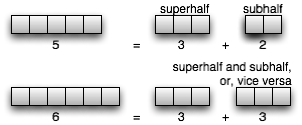For an even number such as 6, the subhalf and superhalf are the same as the half (3). For an odd number such as 5, the subhalf (2) and superhalf (3) differ by one. The subhalf and superhalf of any integer sum to that integer: 3+3=6, and 2+3=5.

Now the first proof. If n is even, take the top half of the triangle and fold it over onto the bottom half. Each row has the same length, because the base increases by one going up, and the flipped top increases by one going down. That length is the width of the original base, plus one from the tip. Since there are n/2 rows, the triangle has the area of a rectangle that is n/2 high and n+1 wide.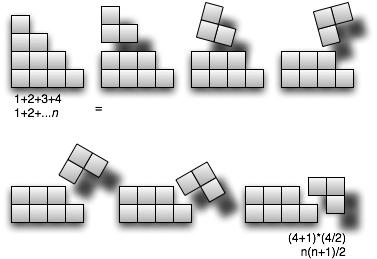For odd n, take the top sub_half of the triangle and fold it onto the bottom _super_half, extending each row except the bottom. The new rectangle is (_n+1)/2 high (the superhalf), but only n wide. Multiply these out, and out the same as the first case.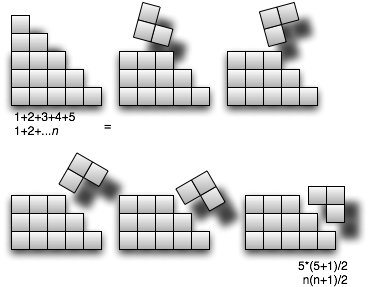A proof that doesn’t require two cases is to use two triangles. Consider the two triangles, which collectively have the area nÃ—(n + 1):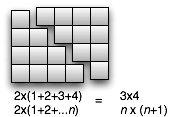This is the same, in algebraic terms, of taking the sum [1+n]+[2+(n-1)]+…+[(n-1)+2]+[n+1]=nÃ—(n+1).

Next week: grounded fractions.